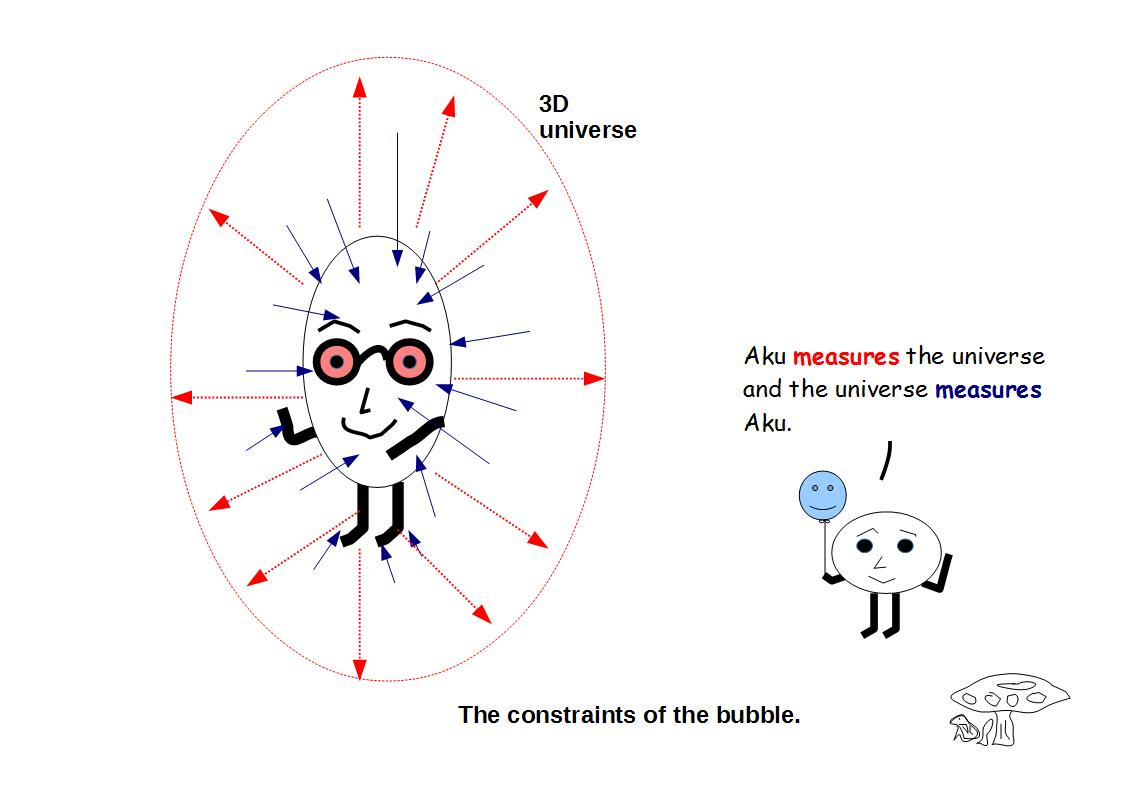# ijspace.org

 b-field and the lower "mass" bound 11th April 2014         Let us first discuss some basic concepts routinely used in vector algebra, and how they apply to the discrete measurement space or j-space.  The finite values of divergence and curl represent the presence of a source and a boundary for a given field.  Let us call this field F.  The divergence and curl of F, being measured as continuous and irrotational are described by the following equations as,                          div F = 0j ,                            curl F = 0j .                                                                       In above equations 0j represents the measured “null” value by the macroscopic observer ObsM in discrete j-space.  However 0j will be measured as finite by Obsi.1     Further the limits of an integral i.e. the interval [0, ∞] measured as an infinite interval by the discrete space observer ObsM, will be measured as a finite interval [0j, ∞j] by Obsi.  The lower and upper bounds are usually determined based on the experimental data as measured by ObsM.      Similarly the infinitesimal period used for differentiation in j-space may contain multiple PE1j events measured by Obsi, which means that there will be stable structures below the measurement threshold of discrete space observer ObsM.     We also note that two observables A and B, measured as commuting with each other i.e. [A, B] = 0 by the observer ObsM in discrete j-space, will be measured as [A, B] = 0j, by Obsi and hence non-commuting with each other.  Therefore a symmetry property exists for the observers of finite capabilities in discrete j-space, but it has no significance for Obsi who has infinite capability.      In other words the assumption of a reference observer with identical capability to ObsM to define an origin, leads to the concept of (VTS) symmetry in discrete j-space.  This assumption is equivalent to defining a lower limit for an interval ( ~quantum), per measurements made in discrete j-space.  We also note that symmetry defined by an observer of lower capability will not necessarily exist for an observer of higher capability, but the opposite must hold.         The arguments presented above, are independent of dimensions of the representation or the number of variables being measured in discrete j-space and they can be generalized to any representation (unlimited number of dimensions or variables), in discrete j-space.   We will also like to point out that definition of 0j as used in classical picture, is invalid in discrete measurement space.  We can not take the differentiation operation for granted, just by replacing real operators by complex operators.   The information contained within 0j is different for real number space than that for complex number space.     We can now consider the condition for b-field given as,The macroscopic observer ObsM with its limited capability, will measure the R.H.S. of the above equation as "0" or null, whereas Obsi will measure R.H.S. as "0j" or finite.  The b-field for ObsM, will appear to be source-free and follow commutation rules, however per Obsi criterion the b-field exists due to a source which can not be physically measured by ObsM.      The commutation property observed by ObsM will be measured as anti-commutative by Obsi, and hence it will give rise to the Lie Bracket in the description provided by ObsM.      The lower mass bound is due to the limitation on the observer's (ObsM) capability.   The time-axis and space-axes will not converge to "origin" simultaneously without the assumption of the symmetry i.e. the time is not necessarily zero when the space is.  We can rephrase it by saying that time and space for a finite capability observer ObsM, do not start simultaneously unless a symmetry is assumed.  We will discuss this rather important point in detail a little later on.  ___________________ 1. Similar concept exists in complex numbers in the form of quaternions, where a quaternion Q is described as,                                  q = scalar + ax + by + cz. When performing normal vector algebra we disregard the "scalar" part, and compute only a, b, and c, the scalars associated with the unit vectors x, y, and z respectively.  Note the j-space can not be assumed to be described as a complex space or any other space in that matter, unless the null bracket {} or 0j for the intended description, is precisely defined.  Another important point to note is that in j-space, the "scalar" part for the quaternion q corresponds to a information space higher than the information space corresponding to the scalars associated, with the unit vectors x, y, and z.Information on www.ijspace.org is licensed under a Creative Commons Attribution 4.0 International License.